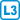## A more difficult assertion about series

Show that if the series $$\displaystyle \sum_{n=1}^{\infty}a_n$$ with positive terms diverges and $$\displaystyle s_n=\sum_{k=1}^{n}a_k$$ is the sequence of partial sums, then the series $$\displaystyle \sum_{n=1}^{\infty}\frac{a_n}{s_n}$$ diverges and the series $$\displaystyle \sum_{n=1}^{\infty}\frac{a_n}{s_n^2}$$ converges.

• #### Hint

For divergence use the Bolzano-Cauchy criterion.

For convergence, write $$a_n=s_n-s_{n-1}$$.

• #### Resolution

Let $$\displaystyle b_n=\sum_{k=1}^n \frac{a_n}{s_n}$$.

Then $$b_m-b_n= \frac{a_{n+1}}{s_{n+1}}+\frac{a_{n+2}}{s_{n+2}}+…+\frac{a_{m}}{s_{m}}\ge \frac{a_{n+1}}{s_{m}}+\frac{a_{n+2}}{s_{m}}+…+\frac{a_{m}}{s_{m}}= \frac{s_m-s_n}{s_m}=1-\frac{s_n}{s_m}$$

But because $$\displaystyle \lim_{n\to\infty} s_n=\infty$$, for every $$n$$ we can choose a sufficiently large $$m$$ such that $$s_m\ge 2s_n$$.

But then $$b_m-b_n\ge\frac12$$, so the series $$\displaystyle \sum_{n=1}^{\infty}\frac{a_n}{s_n}$$ diverges.

If we omit the first term of the series, we obtain the estimate

$$\displaystyle \sum_{n=2}^{\infty}\frac{a_n}{s_n^2} = \sum_{n=2}^{\infty}\frac{s_n-s_{n-1}}{s_n^2} \le \sum_{n=2}^{\infty}\frac{s_n-s_{n-1}}{s_ns_{n-1}} = \sum_{n=2}^{\infty}\left(\frac1{s_{n-1}}-\frac1{s_{n}}\right)=\frac1{a_1}$$

So the series $$\displaystyle \sum_{n=1}^{\infty}\frac{a_n}{s_n^2}$$ converges by the comparison test.